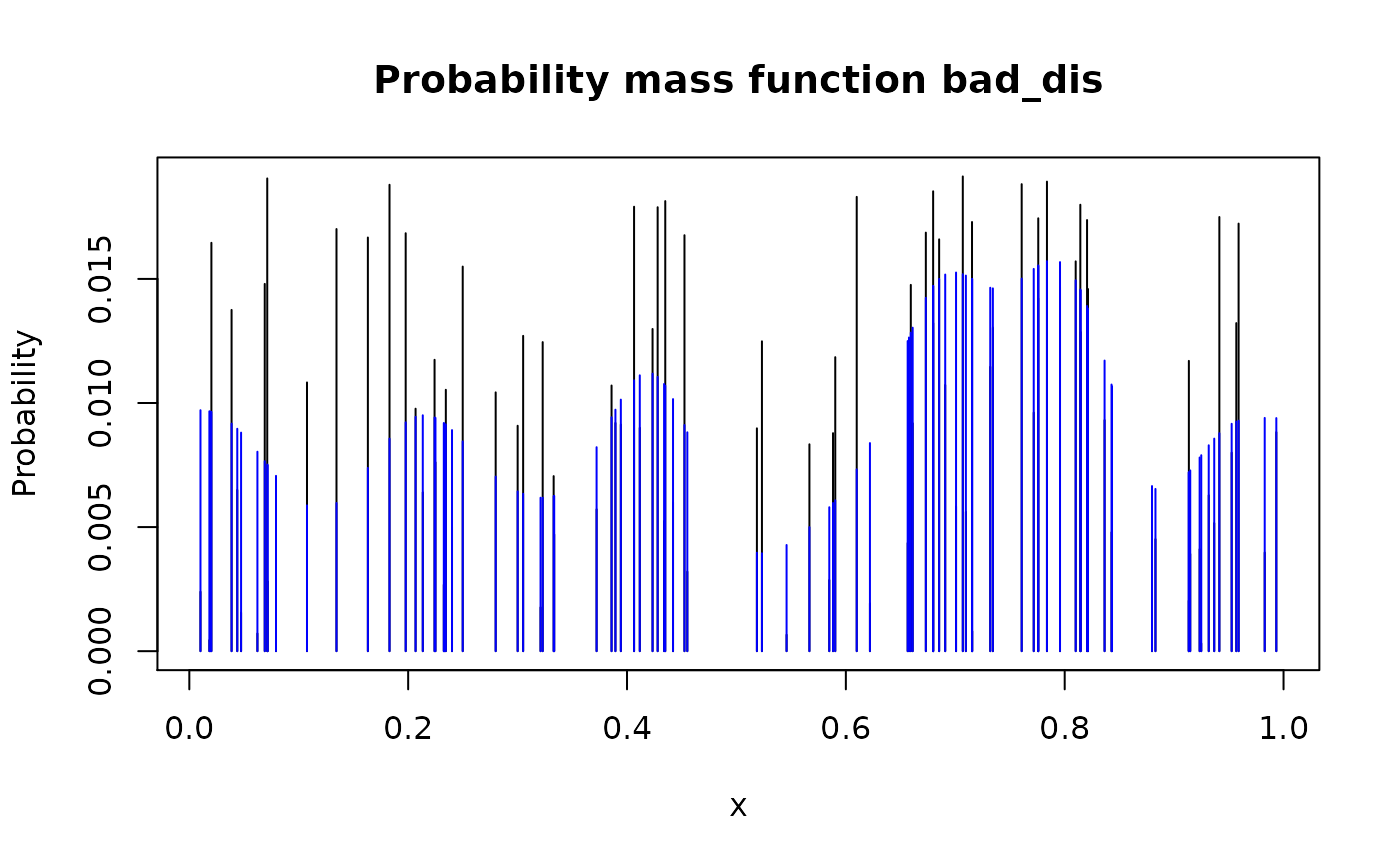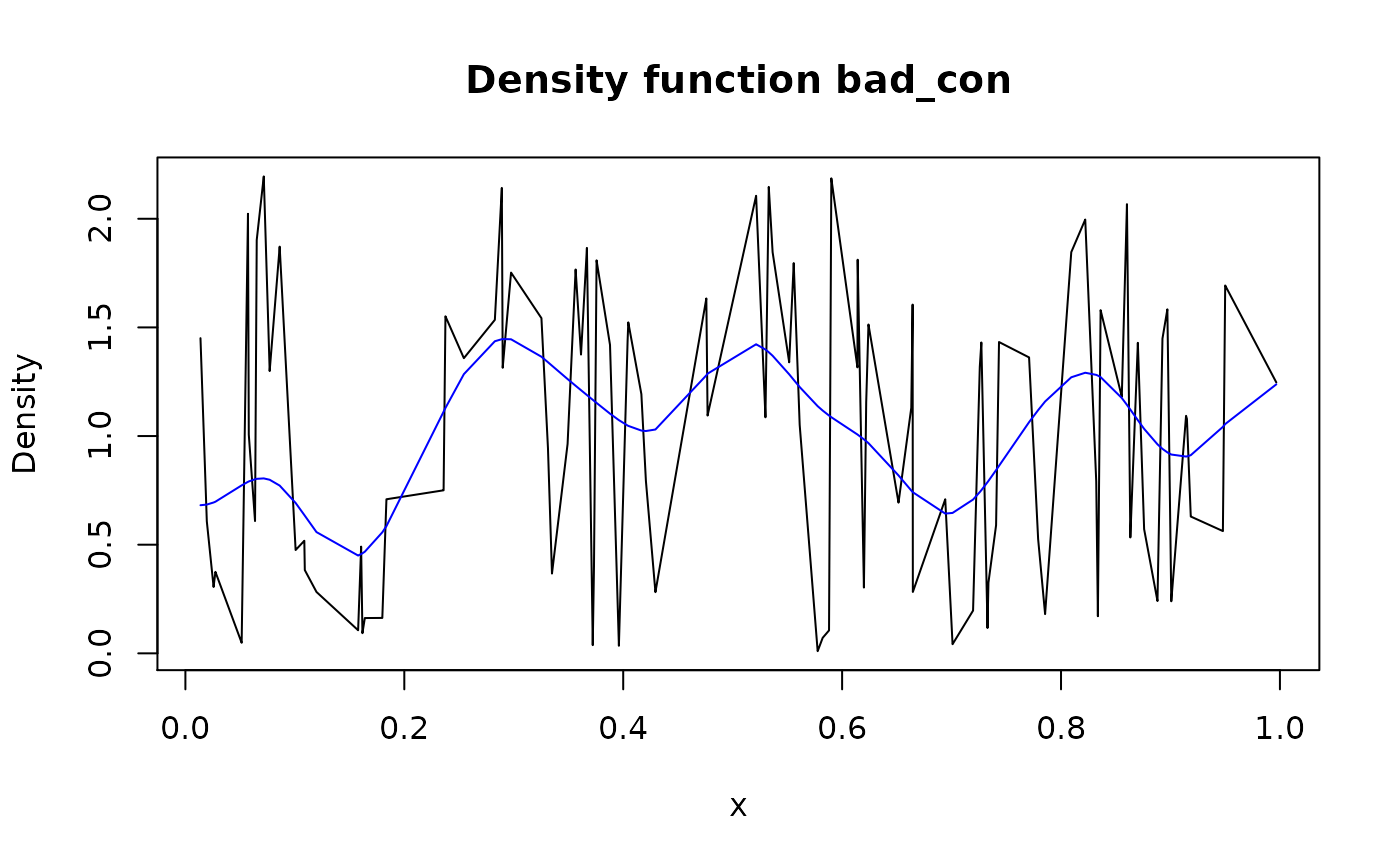Smooth pdqr-function using random sampling and corresponding new_*() function.

form_smooth(f, n_sample = 10000, args_new = list())

## Arguments

f A pdqr-function. Number of elements to sample. List of extra arguments for new_*() to control density().

## Value

A smoothed version of f with the same class and type.

## Details

General idea of smoothing is to preserve "sampling randomness" as much as reasonably possible while creating more "smooth" probability mass or density function.

At first step, sample of size n_sample is generated from distribution represented by f. Then, based on the sample, "continuous" d-function is created with new_d() and arguments from args_new list. To account for density()'s default behavior of "stretching range" by adding small tails, support of d-function is forced to be equal to f's support (this is done with form_resupport() and method "reflect"). Output represents a "smooth" version of f as d-function.

Final output is computed by modifying "y" or "prob" column of f's "x_tbl" metadata to be proportional to values of "smooth" output at corresponding points from "x" column. This way output distribution has exactly the same "x" grid as f but "more smooth" nature.

Other form functions: form_estimate(), form_mix(), form_regrid(), form_resupport(), form_retype(), form_tails(), form_trans()

## Examples

set.seed(101)

# Type "discrete"
plot(bad_dis)lines(smoothed_dis, col = "blue")plot(bad_con)lines(smoothed_con, col = "blue")